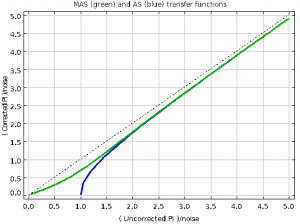# New de-biasing method for POL2 data

Calculation of the polarised intensity (PI) and percentage polarisation (P) values from measurements of I, Q and U usually need to be corrected to take account of the bias towards larger values caused by the presence of noise (particularly noticeable  in areas of low signal-to-noise). This bias occurs because of the squaring of Q and U involved in calculating PI:

PI = √( Q2 + U2 )

Thus both positive and negative Q and U values give a positive PI value, pushing the mean PI value upwards. A correction for this effect is applied if the parameter setting “debias=yes” is specified when running the SMURF pol2map command (note, the default is “debias=no“). To date, this correction has used the so-called “asymptotic estimator” (AS):

PIAS = √( Q2 + U2 – σ2 )

where σ2 is the weighted mean of the variances on Q and U:

σ2 = ( Q2Q2 + U2U2 ) / ( Q2 + U2 )

However, this estimator has the problem that it becomes undefined when

Q2 + U2 < σ

The usual practice is to use an exact value of zero for the PI at such points. However, this can upset the PI noise statistics in very low SNR regions.

However, there are other forms of de-biasing that avoid this problem, such as the “modified asymptotic estimator” (MAS) described by Plaszczynski et al  (MNRAS 2014).   The de-biased MAS estimate of PI is defined by

PIMAS = PI – 0.5*σ2*( 1 – e-(PI/σ)2)/PI

where PI is √( Q2 + U2 ). The PIMAS value is defined for all non-zero PI. The following figure shows PIAS  (blue) and PIMAS (green) plotted against uncorrected PI, assuming σ= 1:The MAS estimator may now be used in pol2map by adding “debiastype=mas” to the pol2map command line. Note, the default de-biasing method is still the asymptotic estimator. Using the MAS estimator may facilitate investigation of PI noise statistics in low SNR areas.